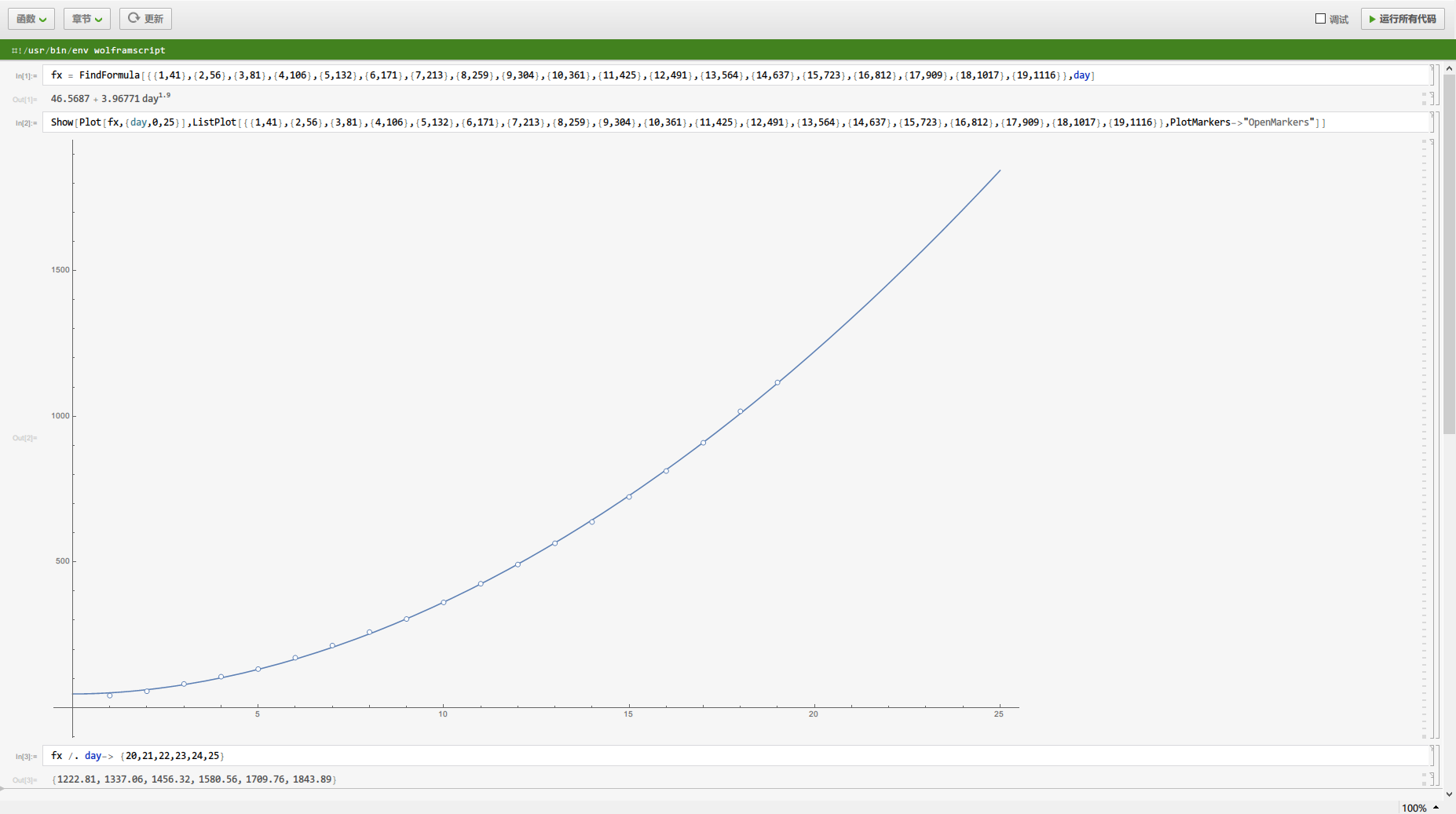# [教程]利用Wolfram Mathematica求拟合

### 软件安装

*安装需要至少10GB可用空间

#### 激活### 使用*注意使用此软件时若输入中文会卡死闪退，建议关闭输入法使用

FindFormula[data,x]

`{1,41},{2,56},{3,81},{4,106},{5,132},{6,171},{7,213},{8,259},{9,304},{10,361},{11,425},{12,491},{13,564},{14,637},{15,723},{16,812},{17,909},{18,1017},{19,1116}`

`fx = FindFormula[{{1,41},{2,56},{3,81},{4,106},{5,132},{6,171},{7,213},{8,259},{9,304},{10,361},{11,425},{12,491},{13,564},{14,637},{15,723},{16,812},{17,909},{18,1017},{19,1116}},day]`

`Show[Plot[fx,{day,0,25}],ListPlot[{{1,41},{2,56},{3,81},{4,106},{5,132},{6,171},{7,213},{8,259},{9,304},{10,361},{11,425},{12,491},{13,564},{14,637},{15,723},{16,812},{17,909},{18,1017},{19,1116}},PlotMarkers->"OpenMarkers"]]`

`fx /. day-> {20,21,22,23,24,25}`### 结语

Wolfram Mathematica是一个非常好用的科学计算软件，还有很多其他实用的功能可以使用。这里只是举出一个我比较常用的功能作为示例，若各位感兴趣的话可以去看看它的在线文档或是其它的视频教学Scroll Up